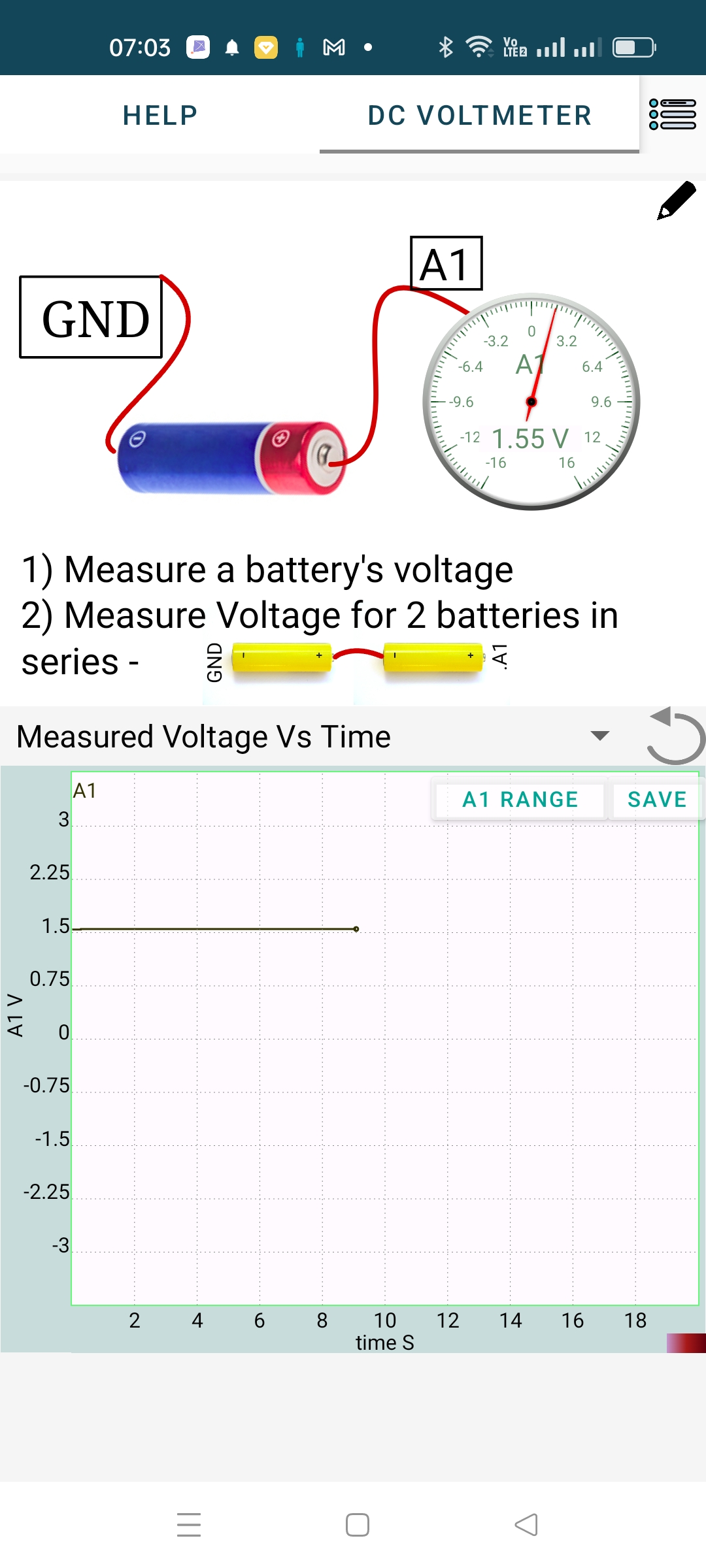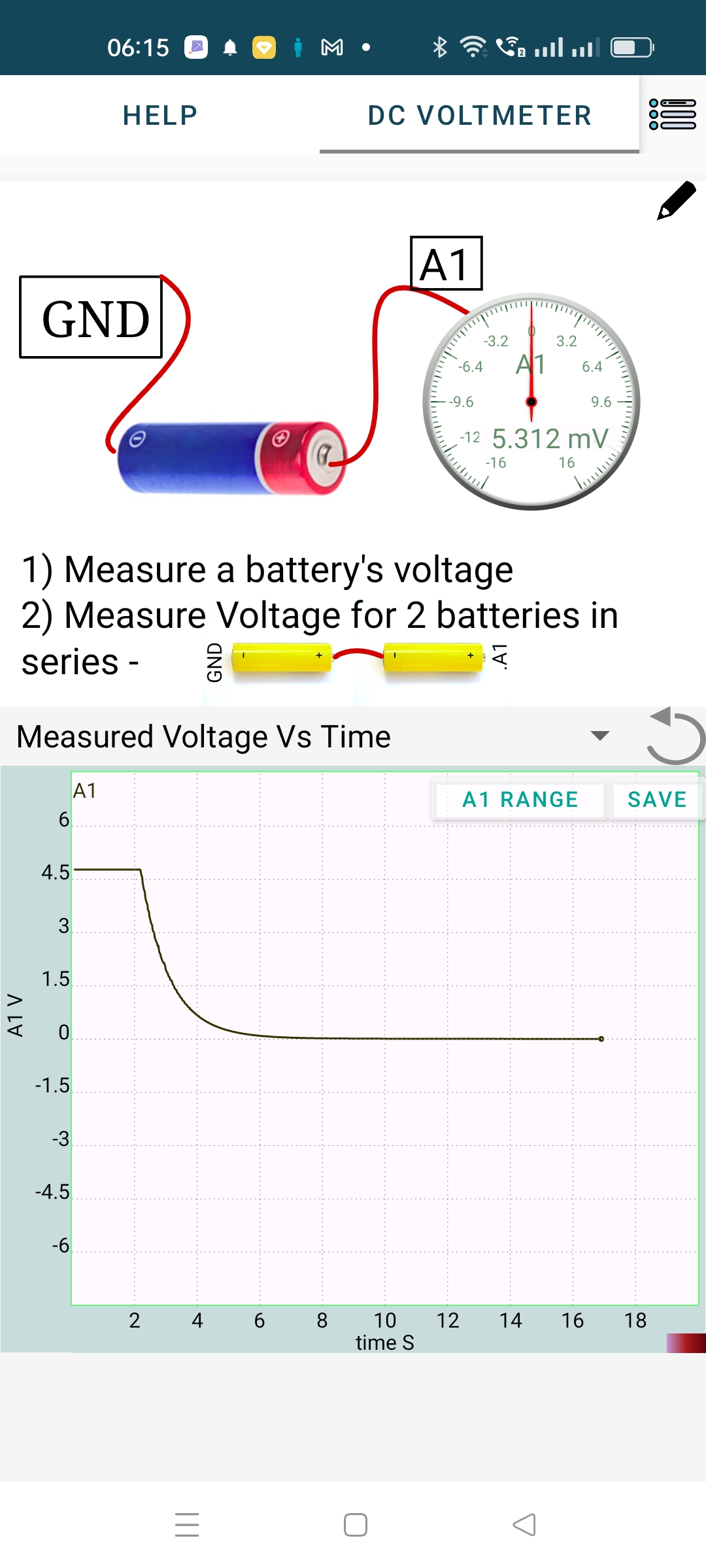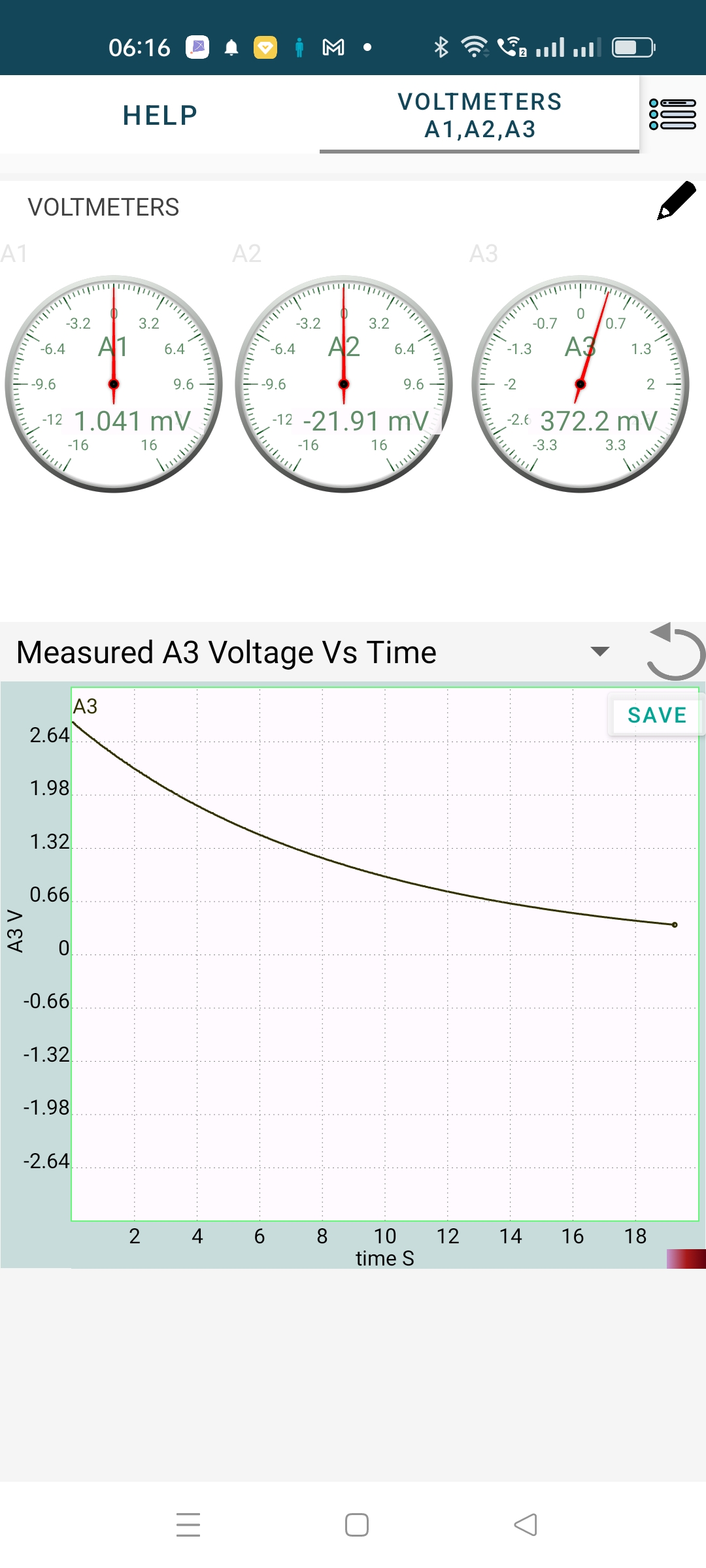# ExpEYES

## Measuring a DC voltage using ExpEYES

### Charge, Current and Voltage

The elecrical charge is a very fundamental property of matter. There are two types of charges in nature, named as positive and negative. Matter contains equal number of opposite charges and therefore electrically neutral. Like charges repel and opposite charges attract. It means that there is push or pull on a charge kept near another charge. Potential or Voltage is a measure of this push. This force is a measure of the energy content of a charge, or the work that can be done by that charge when it moves from a region of higher potential to a lower one. We cannot measure an absolute potential, what we measure is the potential difference between two points. Such a difference can be created by separating the charged particles in matter by chemical reactions, electromagnetic induction etc. Voltage makes the charges move, resulting in the flow of current.

ExpEYES/SEELab3 has several input terminals (A1, A2, A3 etc.) for measuring voltage. Voltage is measured relative to the potential of the ground terminals, considered as at zero volts. If you connect the +ive terminal of the drycell to ground and -ive to A1, the result will be a negative value.

### Procedure

• Connect a DC voltage source (for example, a drycell) between A1 and GND, as shown in the figure below.
• The result is shown on the dial, of A1
• The DC voltage is continuosly measured and plotted against time.

In the PC version you need to tick the checkbox A1 (below Measure Voltages) to display the measured DC value.

The range of the input A1 can be selected. Always set it to a value just above the voltage to be measured. For example, while measuring a 1.5 volt cell the range should be set to 2.5 volts. It will work with the 16 volt range also but the accuracy is better when proper range is selected.

The data of the graph can be saved or shared, in the CSV format. Screenshot also may be saved or shared.Voltage is probably the physical parameter that is measured more than any other. Modern measuring instruments converts all other parameters like temperature, pressure etc. to voltage signals by using appropriate sensor elements.

### Measurement affecting the measured parameter

The very process of measurement may change the value of the measured parameter. A simple example is measuring the temperature of water using a mercury thermometer. Some heat flow happens between water and the thermometer, resulting in changing the temperature of water. This is negligible if the quantity of water is more, but it could be significant for one drop of water.

A similar thing happens while measuring voltage also. Some amount of charge flows into the voltmeter, affecting it’s voltage. An ideal voltmeter should have infinite input impedance, means no current flow into it. Most of the voltmeters have an input impedance of 1 $M\Omega$. It means while measuring a 5 volt source the meter draws 5 micro amperes of current. This will not have any visible effect on voltage sources capable of providing milli Amperes of current.

We can demonstrate the effect of the finite input impedance of the measuring device using a capacitor. Charge a 1 uF capacitor from the 5 volt DC supply and start measurig it using A1. Make the connections as shown in the figure below. Momentarily conenct the capacitor terminal with the loose end of the wire from 5 volt.The voltage as a function of time is shown in the figure on the left below. It can be seen that the voltage goes from 4.7 volts (That’s the supply voltage from USB port of the phone used, may not be exact 5 volts) to zero in a couple of seconds. The 1 uF capacitor losing it’s charge through the input impedance (1 Mohm) of A1 takes that much time.

The figure on the right shows the result using A3 instead of A1. It takes more time because the input impedance of A3 is 10 MOhm. Please not that the measurement by A3 is valid only below 3.3 volts.